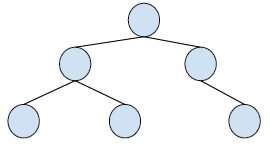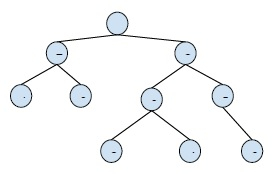# Print common nodes on path from root (or common ancestors) in C++

C++Server Side ProgrammingProgramming

#### C in Depth: The Complete C Programming Guide for Beginners

45 Lectures 4.5 hours

#### Practical C++: Learn C++ Basics Step by Step

Most Popular

50 Lectures 4.5 hours

#### Master C and Embedded C Programming- Learn as you go

66 Lectures 5.5 hours

In this problem, we are given a binary tree and two nodes of the binary tree are defined. And we have to print all the common ancestors of the node i.e. common nodes that occur in the path from traversal from root to the node.

Binary Tree is a special tree whose every node has at max two child nodes. So, every node is either a leaf node or has one or two child nodes.

Example,Ancestor node is a node that is connected to lower-level nodes in a tree.

The common ancestor node of two nodes is a node that is an ancestor node of both nodes in a tree.

For example −In the above Binary tree, we have to find the common ancestor of 0 and 6.

Output − 3, 2

Now, based on this problem, let’s create an algorithm for solving this problem,

## Algorithm

Step 1 : Find the lowest common ancestor of the two nodes of the given tree. And print it.
Step 2 : Traverse upward to the root node and print all the nodes that come in the path.

## Example

Now, let’s create a program to illustrate the solution of this problem,

#include <iostream>
using namespace std;
struct Node {
struct Node* left, *right;
int key;
};
Node* insertNode(int key){
Node* temp = new Node;
temp->key = key;
temp->left = temp->right = NULL;
return temp;
}
struct Node* LowestCommonAncestors(struct Node* root, int n1, int n2){
if (root == NULL)
return NULL;
if (root->key == n1 || root->key == n2)
return root;
Node* left_lca = LowestCommonAncestors(root->left, n1, n2);
Node* right_lca = LowestCommonAncestors(root->right, n1, n2);
if (left_lca && right_lca)
return root;
return (left_lca != NULL) ? left_lca : right_lca;
}
bool printAncestorNodes(struct Node* root, int target){
if (root == NULL)
return false;
if (root->key == target) {
cout << root->key << "\t";
return true;
}
if (printAncestorNodes(root->left, target) ||
printAncestorNodes(root->right, target)) {
cout << root->key << "\t”;
return true;
}
return false;
}
bool printcommonAncestors(struct Node* root, int first, int second){
struct Node* LCA = LowestCommonAncestors(root, first, second);
if (LCA == NULL)
return false;
printAncestorNodes(root, LCA->key);
}
int main(){
Node* root = insertNode(24);
root->left = insertNode(8);
root->right = insertNode(69);
root->left->left = insertNode(12);
root->left->right = insertNode(41);
root->right->left = insertNode(50);
root->right->right = insertNode(3);
root->left->left->left = insertNode(22);
root->right->left->left = insertNode(10);
root->right->left->right = insertNode(6);
if (printcommonAncestors(root, 6, 3) == false)
cout << "No Common nodes";
return 0;
}

## Output

69 24